Home » Subject » Management » Notes » Cost of capital

# Cost of capital

Cost of capital is vital part of investment decision as it is used to measure the value of investment proposal provided by the business concern. It is used as a discount rate to determine the present value of future cash flows related with capital projects. Cost of capital is also termed as cut-off rate, target rate, hurdle rate and required rate of return. When the companies are using different sources of finance, the finance manager must take vigilant decision with regard to the cost of capital; because it is closely associated with the value of the firm and the earning capacity of the firm.

## Concept of Cost of Capital

There is bulk of finance literature to describe this concept. Numerous studies have shown that Cost of capital is the rate of return that a firm must earn on its project investments to maintain its market value and attract funds. It is the required rate of return on its investments which belongs to equity, debt and retained earnings. If a firm fails to earn return at the expected rate, the market value of the shares will fall and it will result in the decrease of overall prosperity of the shareholders. Famous theorist, John J. Hampton described cost of capital as "the rate of return the firm required from investment in order to increase the value of the firm in the market place". Solomon Ezra stated that "Cost of capital is the minimum required rate of earnings or the cut-off rate of capital expenditure" According to James C. Van Horne, Cost of capital is "A cut-off rate for the allocation of capital to investment of projects. It is the rate of return on a project that will leave unchanged the market price of the stock". Another theorist, William and Donaldson explained that "Cost of capital may be defined as the rate that must be earned on the net proceeds to provide the cost elements of the burden at the time they are due".

## Assumption of Cost of Capital

It is documented in theoretical studies that cost of capital is based on some assumptions which are directly related while calculating and measuring the cost of capital. There are three basic concepts:

1. It is not a cost as such. It is merely a hurdle rate.
2. It is the minimum rate of return.
3. It consists of three important risks such as zero risk level, business risk and financial risk.

Cost of capital can be measured with the following equation:Where,
K = Cost of capital.
rj = The riskless cost of the particular type of finance.
f = The financial risk premium.

## Taxonomy of Cost of Capital

Cost of capital may be categorized into the following types on the basis of nature and usage:

1. Explicit and Implicit Cost.
2. Average and Marginal Cost.
3. Historical and Future Cost.
4. Specific and Combined Cost.

1. Explicit and Implicit Cost:
The cost of capital may be explicit or implicit cost on the basis of the computation of cost of capital. Explicit cost is the rate that the firm pays to procure financing. An explicit cost is one that has occurred and is evidently reported as a separate cost. It is defined as direct payment to others in doing business such as wage, rent and materials.
This may be calculated with the following equation;

Where,
CIo = initial cash inflow
C = outflow in the period concerned
N = duration for which the funds are provided
T = tax rate
Implicit cost is the rate of return linked with the best investment opportunity for the firm and its shareholders that will be inevitable if the projects presently under consideration by the firm were accepted. It is the opportunity cost equal to what a firm must give up in order to use factor of production which it already owns and thus does not pay rent for.
Both implicit and explicit costs are actual business cost of firms (Barthwal, 2007).

2. Average and Marginal Cost:
Average cost of capital is the weighted average cost of each element of capital employed by the company. It reflects weighted average cost of all kinds of financing such as equity, debt, retained earnings.
Marginal cost is the weighted average cost of new finance raised by the company. It is the extra cost of capital when the company goes for further raising of finance.

3. Historical and Future Cost:
Historical cost is the cost which is already been incurred for financing a particular project. It is based on the actual cost incurred in the earlier project. Future cost is the expected cost of financing in the proposed project. Expected cost is calculated on the basis of previous experience.

4. Specific and Combine Cost:
The cost of each sources of capital such as equity, debt, retained earnings and loans is termed as specific cost of capital. It is beneficial to determine the each and every specific source of capital. The composite or combined cost of capital is the amalgamation of all sources of capital. It is also called as overall cost of capital. It is used to recognize the total cost associated with the total finance of the company.

## Importance of Cost of Capital

Computation of cost of capital is significant part of the financial management to decide the capital structure of the business concern.
Importance to Capital Budgeting Decision: Capital budget decision mainly depends on the cost of capital of each source. According to net present value method, present value of cash inflow must be more than the present value of cash outflow. Therefore, cost of capital is used for capital budgeting decision.
Importance to Structure Decision: Capital structure is the mix or proportion of the different types of long term securities. Company uses particular type of sources if the cost of capital is suitable. Therefore, cost of capital supports to take decision regarding structure.
Importance to Evolution of Financial Performance: Cost of capital is imperative to determine which affects the capital budgeting, capital structure and value of the firm. It helps to estimate the financial performance of the firm.
Importance to Other Financial Decisions: Cost of capital is also used in some other areas such as, market value of share, earning capacity of securities etc. hence, it plays a major part in the financial management.

Computation of cost of capital:
Computation of cost of capital has two important parts:

1. Measurement of specific costs
2. Measurement of overall cost of capital

## Measurement of Cost of Capital:

It refers to the cost of each specific sources of finance such as:

• Cost of equity
• Cost of debt
• Cost of preference share
• Cost of retained earnings

Cost of Equity: Cost of equity capital is the rate at which investors discount the expected dividends of the firm to determine its share value. Theoretically, the cost of equity capital is described as the "Minimum rate of return that a firm must earn on the equity financed portion of an investment project in order to leave unchanged the market price of the shares".
Cost of equity can be calculated from the following approach:

• Dividend price (D/P) approach.
• Dividend price plus growth (D/P + g) approach.
• Earning price (E/P) approach.
• Realized yield approach.

Dividend Price Approach: The cost of equity capital will be that rate of expected dividend which will maintain the present market price of equity shares.
Dividend price approach can be measured with the following formula: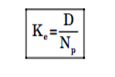Where,
Ke = Cost of equity capital
D = Dividend per equity share
Np = Net proceeds of an equity share
Dividend Price Plus Growth Approach: The cost of equity is calculated on the basis of the expected dividend rate per share plus growth in dividend (R M Srivastava, 2008).
It can be measured by the following formula: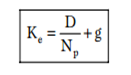Where,
Ke = Cost of equity capital
D = Dividend per equity share
g = Growth in expected dividend
Np = Net proceeds of an equity share
Earning Price Approach: Cost of equity regulates the market price of the shares. It is based on the future earnings forecasts of the equity (R M Srivastava, 2008). The formula for calculating the cost of equity according to this approach is as follows.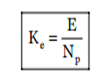Where,
Ke = Cost of equity capital
E = Earnings per share
Np = Net proceeds of an equity share
Realized Yield Approach: It is simple method to compute cost of equity capital (R M Srivastava, 2008). Under this method, cost of equity is calculated byWhere,
Ke = Cost of equity capital.
PVf = Present value of discount factor.
D = Dividend per share.
II. Cost of Debt: Cost of debt is the after tax cost of long-term funds through borrowing. Debt may be issued at par, at premium or at discount and also it may be perpetual or redeemable.
Debt Issued at Par: Debt issued at par means, debt is issued at the face value of the debt. It may be calculated with the following formula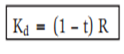Where,
Kd = Cost of debt capital
t = Tax rate
R = Debenture interest rate
Debt Issued at Premium or Discount: If the debt is issued at premium or discount, the cost of debt is calculated with the following formula.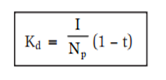Where,
Kd = Cost of debt capital
I = Annual interest payable
Np = Net proceeds of debenture
t = Tax rate
Cost of Perpetual Debt and Redeemable Debt: It is the rate of return which the lenders expect. The debt carries a certain rate of interest.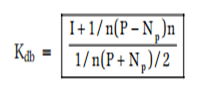Where,
I = Annual interest payable
P = Par value of debt
Np = Net proceeds of the debenture
n = Number of years to maturity
Kdb = Cost of debt before tax
Cost of debt after tax can be calculated with the following formula:Where,
Kda = Cost of debt after tax
Kdb = Cost of debt before tax
t = Tax rate
III. Cost of Preference Share Capital: Cost of preference share capital is the annual preference share dividend by the net proceeds from the sale of preference share. There are two types of preference shares irredeemable and redeemable.
Following formula is used to calculate the cost of redeemable preference share capital:Where,
Kp = Cost of preference share
Dp = Fixed preference dividend
Np = Net proceeds of an equity share
Cost of irredeemable preference share is calculated with the following formula:Where,
Kp = Cost of preference share
Dp = Fixed preference share
P = Par value of debt
Np = Net proceeds of the preference share
n = Number of maturity period.
IV. Cost of Retained Earnings: Retained earnings is one of the sources of finance for investment proposal. It is dissimilar from other sources like debt, equity and preference shares. Cost of retained earnings is the same as the cost of an equivalent fully subscripted issue of additional shares, which is measured by the cost of equity capital.
Cost of retained earnings can be calculated with the following formula:Where,
Kr = Cost of retained earnings
Ke = Cost of equity
t = Tax rate
b = Brokerage cost

Measurement of Overall Cost of Capital:
It is also known as weighted average cost of capital and composite cost of capital. Weighted average cost of capital is the expected average future cost of funds over the long run found by weighting the cost of each specific type of capital by its proportion in the firm's capital structure.

The computation of the overall cost of capital (Ko) involves the following steps.
(a) Assigning weights to specific costs.
(b) Multiplying the cost of each of the sources by the appropriate weights.
(c) Dividing the total weighted cost by the total weights.
The overall cost of capital can be calculated with the following formula;Where,
Ko = Overall cost of capital
Kd = Cost of debt
Kp = Cost of preference share
Ke = Cost of equity
Kr = Cost of retained earnings
Wd= Percentage of debt of total capital
Wp = Percentage of preference share to total capital
We = Percentage of equity to total capital
Wr = Percentage of retained earnings
Weighted average cost of capital is calculated in the following formula also:Where,
Kw = Weighted average cost of capital
X = Cost of specific sources of finance
W = Weight, proportion of specific sources of finance.
To, summarize, cost of return is defined as the return the firm's investors could expect to earn if they invested in securities with comparable degrees of risk. The cost of capital signifies the overall cost of financing to the firm. It is normally the relevant discount rate to use in evaluating an investment. Cost of capital is important because it is used to assess new project of company and permits the calculations to be easy so that it has minimum return that investor expect for providing investment to the company.